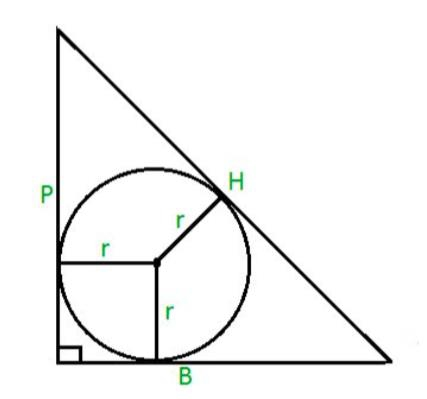# Area of Incircle of a Right Angled Triangle in C Program?

CServer Side ProgrammingProgramming

#### C in Depth: The Complete C Programming Guide for Beginners

45 Lectures 4.5 hours

#### Practical C++: Learn C++ Basics Step by Step

Most Popular

50 Lectures 4.5 hours

#### Master C and Embedded C Programming- Learn as you go

66 Lectures 5.5 hours

To find the area of a circle inside a right angled triangle, we have the formula to find the radius of the right angled triangle, r = ( P + B – H ) / 2.

Given the P, B and H are the perpendicular, base and hypotenuse respectively of a right angled triangle.

Area of a circle is given by the formula,

Area = π*r2

where π = 22 / 7 or 3.14 and r is the radius of the circle.

Hence the area of the incircle will be given by the formula,

Area = π* ((P + B – H) / 2)2.## Example

#include
#define PI 3.14159265
int main() {
float area,P = 3, B = 4, H = 5;
area=(P + B - H) * (P + B - H) * (PI / 4);
printf("Area = %f", area);
return 0;
}

## Output

Area = 3.141593